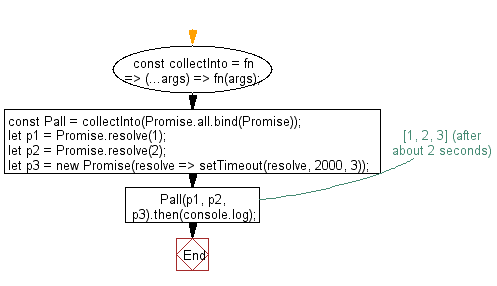# JavaScript: Change function that accepts an array into a variadic function

## JavaScript fundamental (ES6 Syntax): Exercise-43 with Solution

Write a JavaScript program to change function that accepts an array into a variadic function.

Note: Given a function, return a closure that collects all inputs into an array-accepting function.

• Given a function, return a closure that collects all inputs into an array-accepting function.

Sample Solution:

JavaScript Code:

``````//#Source https://bit.ly/2neWfJ2
const collectInto = fn => (...args) => fn(args);
const Pall = collectInto(Promise.all.bind(Promise));
let p1 = Promise.resolve(1);
let p2 = Promise.resolve(2);
let p3 = new Promise(resolve => setTimeout(resolve, 2000, 3));
Pall(p1, p2, p3).then(console.log); // [1, 2, 3] (after about 2 seconds)
```
```

Sample Output:

```[1,2,3]
```

Flowchart:Live Demo:

See the Pen javascript-basic-exercise-1-43 by w3resource (@w3resource) on CodePen.

Improve this sample solution and post your code through Disqus

What is the difficulty level of this exercise?

Test your Programming skills with w3resource's quiz.

﻿

## JavaScript: Tips of the Day

Chunks an array into n smaller arrays

Example:

```const tips_chunkIntoN = (arr, n) => {
const size = Math.ceil(arr.length / n);
return Array.from({ length: n }, (v, i) =>
arr.slice(i * size, i * size + size)
);
}
console.log(tips_chunkIntoN([1, 2, 3, 4, 5, 6, 7,8], 4));
```

Output:

```[[1,2],[3,4],[5,6],[7,8]]
```